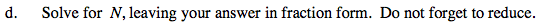### Home > PC > Chapter 8 > Lesson 8.2.3 > Problem8-104

8-104.
1. You may know that 0.33333… is equal to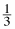. Here is a short proof to help justify the fact. Let N = 0.333333…. Homework Help ✎

1. What does 10N equal?

2. Fill in the blanks: 10N = ____
N
= ____

3. What expression do you get by subtracting the two equations above?

4. Solve for N, leaving your answer in fraction form. Do not forget to reduce.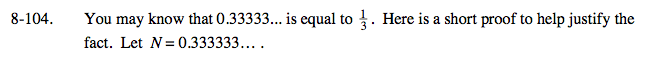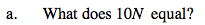3.333...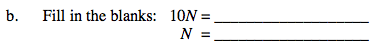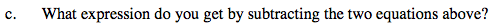9N = 3